StatLect

# Type II error

In hypothesis testing, a Type II error occurs when the null hypothesis is not rejected even though it is false.

The probability of committing Type II errors can be reduced by increasing the sample size and the statistical significance.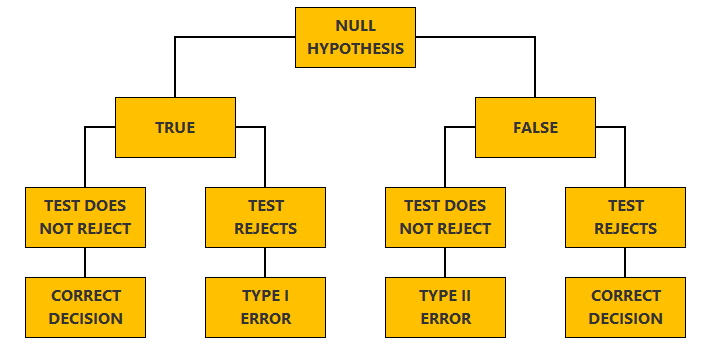## Synonyms

Type II errors are also referred to as errors of the second kind.

## Explanation

When we perform a test of hypothesis, we decide whether the null hypothesis should be rejected or not, based on a test statistic:

• we reject the null hypothesis when the value taken by the statistic belongs to a critical region that has been specified in advance;

• otherwise, we do not reject the null.

This decision process is prone to the following kind of error: we may fail to reject the null hypothesis when it is false.

Errors of this kind are dubbed Type II errors, in order to distinguish them from Type I errors, which occur when the null hypothesis is rejected despite being true.

## Probability of Type II errors

Earlier we mentioned that the outcome of a test of hypothesis depends on the value taken by a test statistic. In turn, this value depends on the data used to compute the statistic.

But the data is random. Thus, before the data is observed, the test statistic can be regarded as a random variable.

As a consequence, the probability that a Type II error occurs is equal to the probability that the realization of a random variable (the test statistic) will not belong to the critical region.

We will explain below that the probability of committing Type II errors can be exactly quantified through a function called power function.

## Example

Suppose that we observe a sample of draws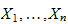from a normal distribution.

The mean of the distributionis known and its varianceis unknown.

### Null hypothesis

We want to test the null hypothesis that the variance of the distribution is equal to: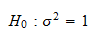### Test statistic

As demonstrated in the lecture on Hypothesis tests about the variance, we can use the Chi-square statistic as a test statistic.

First, we compute the sample variance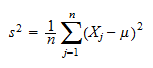Then, we calculate the Chi-square statistic: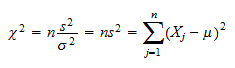where we set, as specified in the null hypothesis.

### Critical value

We check whether the value taken by the Chi-square statistic belongs to a critical region.

The outcome of the hypothesis test is determined as follows:

• if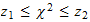, then we do not reject the null hypothesis;

• if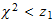or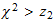, then we reject the null hypothesis.

The two thresholdsandare the critical values of the test.

### Incorrect decision

Suppose that the true varianceis different from.

If, then we fail to reject the null hypothesis.

This is a Type II error! We are not rejecting the null even if it is false.

### Probability of committing Type II errors

In this example, the probability of committing Type II errors is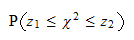As proved here, this probability can be calculated using the distribution function of a Chi-square random variable. However, it depends on the true and unknown variance.

Therefore, the probability of committing a Type II error is not uniquely pinned down, but it depends on the true variance.

The function that links the value of the true variance to the probability of a Type II error is called power function.

## Power

The power of a test is the probability that the test will reject a false null (the higher, the better).

The power depends on which alternative hypothesis is true.

If we denote the probability of committing a Type II error by, then the power is equal to.

In other words:

• if a test has low power, then there is a high probability of committing a Type II error;

• if a test has high power, then there is a low probability of committing a Type II error;

## How to reduce the probability of Type II errors

Usually, power is an increasing function of sample size: the more observations we have, the more powerful the test.

Therefore, we can decrease the probability of Type II errors by increasing the sample size.

Moreover, power is an increasing function of the size of the test (or significance).

As a consequence, we can reduce the probability of Type II errors by increasing the size/significance of the test.

## Want to see the formulae?

If you want to learn more about the relations among power, significance and sample size, you are advised to check the formulae in the lecture on Hypothesis tests about the mean.

## More details

Go to the lecture entitled Hypothesis testing for more details about Type II errors.

Previous entry: Type I error